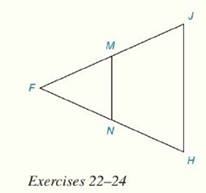Chapter 4.CR, Problem 22CR### Elementary Geometry for College St...

6th Edition
Daniel C. Alexander + 1 other
ISBN: 9781285195698

#### Solutions

Chapter
Section### Elementary Geometry for College St...

6th Edition
Daniel C. Alexander + 1 other
ISBN: 9781285195698
Textbook Problem
3 views

# Review ExercisesIn Review Exercises 22 to 24, M and N are the midpoints of F J - and F H - respectively.Given: Isosceles ∆ F J H with F J - ≅ F H - F M = 2 y + 3 N H = 5 y - 9 J H = 2 y Find: The perimeter of ∆ F M N

To determine

To Find:

The perimeter of an isosceles triangle using the information provided.

Explanation

Theorem on triangle:

The line segment that joins the midpoints of two sides of a triangle is parallel to the third side and has a length equal to one-half the length of the third side.

That is, ABC with midpoints M and N of AB- and AC-, respectively. Then MN-BC-. It can be concluded that BC=12(MN)

Calculation:

It is given that FJH is an isosceles triangle with M and N are the midpoints of FJ- and FH- respectively.

Also given that FJ-FH-, FM=2y+3, NH=5y-9 and JH=2y.

The perimeter of FMN is FM+MN+FN.

Since FJ-FH- and M and N are the midpoints of FJ- and FH-, FN=NH=FM=MJ

### Still sussing out bartleby?

Check out a sample textbook solution.

See a sample solution

#### The Solution to Your Study Problems

Bartleby provides explanations to thousands of textbook problems written by our experts, many with advanced degrees!

Get Started